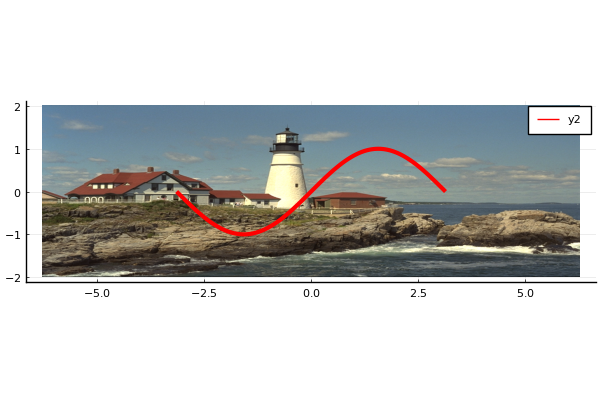# Images with custom axesusing Plots
pyplot()
using TestImages
img = testimage("lighthouse")
plot([-π, π], [-1, 1], reverse(img, dims = 1), yflip = false, aspect_ratio = :none)
plot!(sin, -π, π, lw = 3, color = :red)